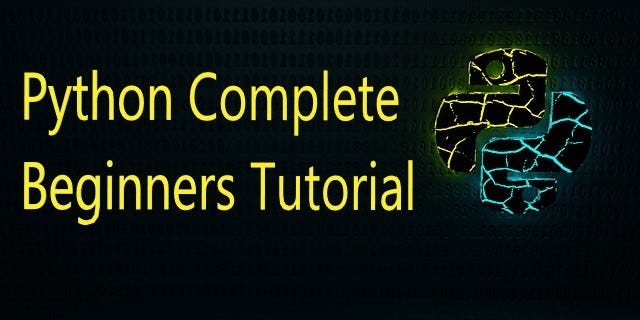# Python Complete Beginners Tutorial | PART-2Python Complete Beginners Tutorial. Introduction To Python | Function, Recursion, Type conversion and Coercion, Lambda Function, Strings.

Introduction To Python | Function, Recursion, Type conversion and Coercion, Lambda Function, Strings

### Functions In Python

Function is a group of Inter-related statements that perform a specific task. Functions help as to break large programs into smaller blocks or modular chucks.

### Types of Functions

a) Built ­in functions -­ Functions that are built within Python by default.

*b) User defined functions *-­ Functions that are defined by the users.

### Built ­in Functions

Mathematical Function

``````#Example 2.1
import math           #import math module
math.pow(var1,var2)
math.sqrt(var1)
math.sin(var1)
math.cos(var1)
math.log10(var1)
... etc``````

String Function

``````#Example 2.2
len(),lower(),upper(),find(),count(),replace(),strip()... etc``````

## Python Programming Tutorials For Beginners

Python Programming Tutorials For Beginners

## Python Complete Beginners Tutorial | PART-1

Python Complete Beginners Tutorial, python has simple easy-to-use syntax. This makes Python an excellent language to learn to program for beginners.

## Python Programming: A Beginner’s Guide

Python is an interpreted, high-level, powerful general-purpose programming language. You may ask, Python’s a snake right? and Why is this programming language named after it?

## Guide to Python Programming Language

Guide to Python Programming Language

## Python Hello World Program - Create & Run Your First Python Program in PyCharm

Python Hello World Program - Your first step towards Python world. Learn how to create the Hello World Python program in PyCharm.